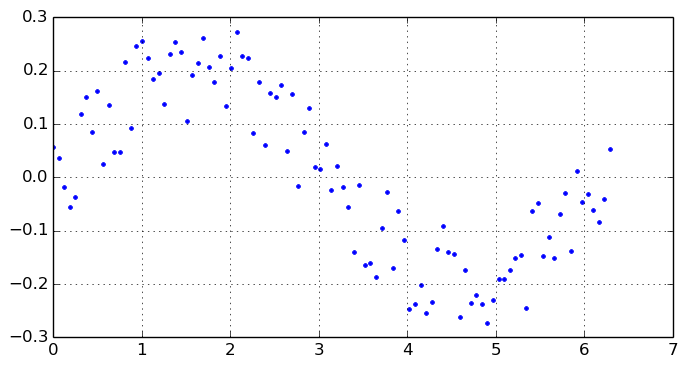## Calling Python (and vice versa)

### Calling Python from Julia

Julia provides several API interfaces to Python in different packages:

• PyCall -- low-level interface to Python
• PyPlot -- plotting library Matplotlib
• Pandas -- front end to Pandas library
• Sympy -- symbolic mathematics library sympy
• [ ... YT -- astrophysical package yt ... ]
In :
using PyCall

@pyimport math
@pyimport numpy as np

In :
math.sin(math.pi) - sin(pi)

Out:
0.0

In :
n = pyeval("2^50")
# typeof(n)

Out:
48


Interestingly, Julia arrays are wrapped as NumPy array and shared, not copied.

In :
A = rand(4, 4)

Out:
4x4 Array{Float64,2}:
0.581857   0.507183  0.230739   0.309339
0.541774   0.838642  0.828332   0.550917
0.0760299  0.714324  0.0540574  0.355525
0.60623    0.299238  0.432295   0.963589

In :
Apy = PyObject(A)

Out:
PyObject array([[ 0.58185661,  0.50718263,  0.23073862,  0.30933888],
[ 0.54177354,  0.83864155,  0.82833172,  0.55091736],
[ 0.07602986,  0.71432411,  0.05405742,  0.3555251 ],
[ 0.6062302 ,  0.29923772,  0.43229519,  0.96358937]])

In :
# det(Apy)    # not possible, no method det(::PyObject)
np.det(A)     # or: det(convert(Float64, Apy))

Out:
-0.1638918638620552

In :
@pyimport scipy.linalg as la    # @pyimport scipy.linalg not possible (why?)
Ainv = la.inv(A)

Out:
4x4 Array{Float64,2}:
2.47741   -0.581389  -1.04385   -0.0777786
0.383502   0.160237   1.21766   -0.663995
-1.27247    1.73184   -1.0481    -0.19495
-1.10686   -0.460945   0.748797   1.38038

In :
A[1, 1] = 0.0;
Apy

Out:
PyObject array([[ 0.        ,  0.50718263,  0.23073862,  0.30933888],
[ 0.54177354,  0.83864155,  0.82833172,  0.55091736],
[ 0.07602986,  0.71432411,  0.05405742,  0.3555251 ],
[ 0.6062302 ,  0.29923772,  0.43229519,  0.96358937]])


Example: Root finding for a difficult and well-known test function

In :
fn(x) = log(x) + x*x/(2.0*exp(1.0)) - 2.0*x/sqrt(exp(1.0)) + 1.0

Out:
fn (generic function with 1 method)


This test function is so flat between 1.0 and 3.4 that every root finder except perhaps an arbitrary precision one will not be able to locate this root exactly.
The true root is obviously sqrt(e) = 1.6487212707001282.

In :
# Pkg.add("Roots")
using Roots

In :
fzero(fn, 1.0, 3.4)

Out:
1.6487190246582024

In :
@pyimport scipy.optimize as spo  # 1-dim: brentq, brenth, ridder, bisect, newton
spo.brentq(fn, 1.0, 3.4)

Out:
1.6487065682417044


Example: Interpolation and integration

In :
using HDF5


HDF5

In :
# h5write("xydata.h5", "xy", [x y])
xy = h5read("xydata.h5", "xy");
x = xy[1:101, 1]; y = xy[1:101, 2];


Imagine there is no good enough spline interpolation available in Julia. We utilize the 'interpolate' module in SciPy and do the integration in Julia.

In :
@pyimport scipy.interpolate as si

f2 = si.interp1d(x, y, kind="cubic")

# f2(xx) ? f2 not recognized as 'callable'
# pycall(f2, Float64, pi)

Out:
PyObject <scipy.interpolate.interpolate.interp1d object at 0x7f2748073510>


f2 is a Python object, to evaluate we need to apply pycall to it.
We visualize the data points and the spline interpolation.

In :
xx = linspace(0, 2*pi, 1000);
yy = pycall(f2, PyAny, xx);

In :
using PyPlot
figure(figsize = (8.0, 4.0))
# plot(xx, yy, "r")
plot(x, y, "b.")
grid()

Warning: imported binding for transpose overwritten in module __anon__
INFO: Loading help data...In :
@pyimport scipy.integrate as spq

In :
spq.quad(f2, 0.0, pi)

Out:
(0.425203989888956,9.573535663910472e-6)


Instead, we transform this interpolated Python function back to Julia and integrate it here using a Gauss-Kronrod quadrature.

In :
f22(x) = pycall(f2, PyAny, x)

quadgk(f22, 0, pi)

Out:
(0-dimensional Array{Float64,0}:
0.425204,6.025219920562742e-9)


Example: Gauss' Hypergeometric Function

Julia does not have an implementation of the Hypergeometric function 2F1, but Python has.

In :
@pyimport scipy.special as special

println(special.hyp2f1(1/3, 2/3, 5/6, 27/32))  # 8/5
println(special.hyp2f1(1/4, 1/2, 3/4, 80/81))  # 9/5

/usr/lib/python2.7/dist-packages/scipy/integrate/quadpack.py:295: UserWarning: The maximum number of subdivisions (50) has been achieved.
If increasing the limit yields no improvement it is advised to analyze
the integrand in order to determine the difficulties.  If the position of a
local difficulty can be determined (singularity, discontinuity) one will
probably gain from splitting up the interval and calling the integrator
on the subranges.  Perhaps a special-purpose integrator should be used.
warnings.warn(msg)


1.599999999999999
1.7999999999999983



These are two of the very few points where the hypergeometric function takes on rational values.
Unfortunately, the Python hypergeometric function is spuriously wrong (not inaccurate, but totally wrong) in the complex plane!

#### SymPy

In :
using SymPy

In :
x, y, z = Sym(:x, :y, :z)  # or Sym("x y z")

Out:
(x,y,z)

In :
limit(sin(x)/x, x, 0.0)

Out:
$$1$$
In :
z = diff(sin(x)/x, x)

Out:
$$\frac{1}{x} \cos{\left (x \right )} - \frac{1}{x^{2}} \sin{\left (x \right )}$$
In :
z = integrate(sin(x)/x, x, 1, Inf)

Out:
$$- \operatorname{Si}{\left (1 \right )} + 1.5707963267949$$
In :
float(z)

Out:
0.6247132564277136


### Excursion: Calling C from Julia

Julia has a built-in feature to call functions in external shared libraries (C API). The syntax is
ccall((function symbol, library name) return type, (argument types), arguments...)

In :
c_sin(x) = ccall((:sin,"libm"), Cdouble, (Cdouble,), x)

Out:
c_sin (generic function with 1 method)

In :
c_sin(pi/4)

Out:
0.7071067811865475

Warning: imported binding for transpose overwritten in module __anon__
Warning: using SymPy.n in module Main conflicts with an existing identifier.



The problem is that this approach will not make functions, defined in Julia, available to the C code.
See the "Rcpp" discussion in R.

### Calling Julia from Python

The pyjulia Python module is developing an interface to Julia that works with Python 2 & 3. (pyjulia is still in an experimental phase).

import julia
j = julia.Julia()

j.eval("2^10")                  #=> 1024
j.eval("2^100")                 #=> 0
j.eval("big(2)^100")            #=> 1267650600228229401496703205376L

j.sqrt(2.0)                     #=> 1.4142135623730951
j.help("sqrt")
# Base.sqrt(x)

#  Return \sqrt{x}. Throws "DomainError" for negative "Real"
#  arguments. Use complex negative arguments instead. The prefix
#  operator "√" is equivalent to "sqrt".

from julia import randn as r
r(100)

# works with julia and user-defined packages
import numpy as np
x = np.array([0.0, 1.0])

from julia import NumericalMath
NumericalMath.trapz(x, x)
# 0.5